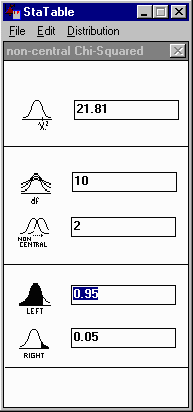Stat TablesStaTable (pronounced Stat-Table) is a compact program that runs on the family of IBM PC compatible computers under Microsoft Windows. It is a free program from Cytel Software, the makers of Stat Exact, a solution for categorical data analysis. This uility enables you to look up both tail areas and percentage points of 25 statistical distributions. You can read off a tail area or a percentage point of a random variate simply by typing a number into a pop-up window; no interpolation, no calculating errors, no hunting for tables. Continuous distributions available are: Normal, Uniform, Triangular, Beta, Gamma, Weibull, Chi-square, Non-central Chi-square, Student's T, Non-central Student's T, F-distribution, Non-central F, Gumbel, Logistic, Exponential, Lognormal, Pareto, Cauchy, and Bivariate Normal. Discrete distribtions are Binomial, Poisson, Geometric, Hypergeometric, Multinomial, and Negative Binomial The program is distributed as a .ZIP file and is password protected (get Password here). The command line for pkunzip is pkunzip -s"Exact Password" statable. Download statable.zip here. Web-based version for you Mac users:StatTables